# UFR 4-18 Evaluation

(diff) ← Older revision | Latest revision (diff) | Newer revision → (diff)

# Evaluation

## Comparison of CFD Calculations with Experiments

### Global comparisons

#### Pressure loss coefficient

The following table gives the numerical values of the presure loss coefficient in Ames et al. experiment as well as those obtained in the main computations. Note that the experimental data shown here have been sent by the authors of the experiment and are different from the ones found in Ames et al. (2005).

Figure 25 shows these values and compares them to existing correlations from the literature (note that Ames et al. experimental values are very close to Metzger et al. correlation):

• Metzger et al. (1982) :

for $Re_{D}<10^{4}$, $\displaystyle f = 0.317 {Re_D}^{−0.132}$ , for $Re_{D}>10^{4}$, $\displaystyle f = 1.76 {Re_D}^{−0.318}$

• Jacob (1938) :

$f=(0.25+0.1175/(P/D-1)^{1.08})Re^{-0.16}$Table 5 compares various comptations to the experiment and gives the relative errors. Large Eddy Simulation shows a very good agreement with the correlations and Ames et al. experimental results. The relative error is equal to 1% end 3% for the two computed Reynolds numbers, respectively. The EB-RSM model shows a very satisfactory pressure loss coefficient at $Re_{D}=10000$(1% error) but the result is worse at $Re_{D}=30000$(10% error). The kω-SST model shows globally good results (7% and 6% errors) and the φ-model poorer results with an error of 17% and 12% for the two Reynolds numbers, respectively.

Wall resolved LES is therefore a good candidate to obtain satisfactory pressure loss coefficients in the present configuration. The EB-RSM model gives very good results at the lowest Reynolds number but a substantial error is observed at $Re_{D}=30000$. Although the kω-SST model exhibits pretty good results, the physics of the present flow is not well captured as it will be shown later and one can't rely on this model. The φ-model gives the worst results and is not reliable.

Table 5: Pressure loss coefficient values for the different computations compared to Ames et al. experimental values.
Ames et al. $Re_{D}=3000$LES3000 Ames et al. $Re_{D}=10000$LES10000 EBRSM10000 PHI10000 kwSST10000 Ames et al. $Re_{D}=30000$EBRSM30000 PHI30000 kwSST30000
Value 0.111 0.1076 0.095 0.0939 0.0963 0.0789 0.0883 0.07 0.0771 0.0615 0.0744
Error (%) - 3 - 1 1 17 7 - 10 12 6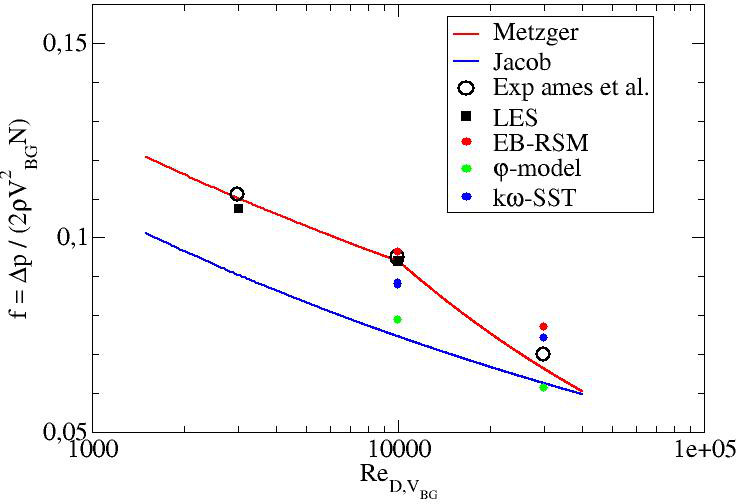Figure 25: Pressure loss coefficient

#### Average Nusselt Number

Table 6 gives the numerical values of the average Nusselt numbers on the bottom wall in Ames et al. experiment as well as those obtained in the main computations (go to "Test Case Studies" section to find the definition of the Nusselt number and its average on the bottom wall).

Figure 26 shows these values and compares them to existing correlations from the literature (Note again that the Ames et al. experimental results are close to the Metzger correlation):

• Metzger et al. (1982) :

$Nus=0.079Re^{0.717}$• Van Fossen (1982) :

$Nus=0.153*Re^{0.686}$Note that the local experimental results have an error of +/- 12%, +/-11.4%, and +/-10.5% for the 3 000, 10 000, and 30 000 Reynolds numbers, respectively, in the endwall regions adjacent to the pins and +/- 9% away from the pin.

Table 6 compares the computations with the experiment and gives the relative errors. One can see that the two models using an eddy viscosity (φ-model and kω-SST) are too far from the experimental results, except the φ-model at $Re_{D}=30000$. However, as it will be shown later, this model exhibits wrong physics. LES and EB-RSM yield very satisfactory values in the error interval. The superiority of these two models will be discussed later when dealing with the local normalized Nusselt number on the bottom wall. Ames and Dvorak (2006a) used standard, Realizable k-ε and RNG k-ε models. They showed a substantial underestimation of the average Nusselt number when the pins were heated. Delibra et al. (2009) used the URANS ζ-f model (very close to the φ-model utilized in the present work) at the 10000 and 30000 Reynolds numbers. They obtained average Nusselt numbers of 46.2 and 122.3, respectively. These values are further from the experimental ones. The temperature was imposed at the wall in Delibra et al. (2009). Imposing the temperature at the wall in the present work with the φ-model led to average Nusselt numbers of 41 and 100, respectively. This shows that imposing the temperature gives higher Nusselt numbers than the ones obtained by imposing the heat flux with this model. The effect of the temperature boundary condition has also been tested with the LES at $Re_{D}=10000$and the EB-RSM model at $Re_{D}=30000$. The average Nusselt numbers were equal to 46 and 115.2, respectively. No clear conclusion can be drawn for these two models which give a more realistic physics than the ones using an eddy viscosity concerning the effect of imposing the temperature instead of the heat flux at the bottom wall. However, one can state that there is a difference between the two methods. Delibra et al. (2008) also reported LES computations. The one carried out for $Re_{D}=30000$was too coarse with a short time integration and will not be considered here. LES at $Re_{D}=10000$gave an average Nusselt number equal to 44.3 which is lower than the one obtained in the present work even with a fixed temperature at the wall. The error compared to the experimental value is equal to 18% which is too high. This discrepancy can be attributed to two factors:

• the mesh was probably still too coarse, not in the wall normal directions but in the stream-wise and span-wise ones (the mesh in the present study contains 76 million cells and their mesh contained 5.5 million cells),
• the boundary condition using an imposed temperature is less representative of the experiment.

Finally, Delibra et al. (2010) switched to use a hybrid RANS/LES approach to overcome the understimation of the Nusselt number but the results where not much better than the ones obtained with the URANS approach based on the ζ-f model.

Table 6: Average Nusselt number on the bottom wall
Ames3000 LES3000 Ames10000 LES10000 DLES10000(*) EBRSM10000 PHI10000 kwSST10000 DURANS10000(*) Ames30000 EBRSM30000 PHI30000 kwSST30000 DURANS30000(*)
Value 22.1 21.6 54.1 48.6 44.1 48.1 37.6 33.1 46.2 111.5 114.8 93.3 72 122.3
Error (%) - 2 - 10 18 11 30 39 14 - 3 16 35 9

(*) Delibra et al. results.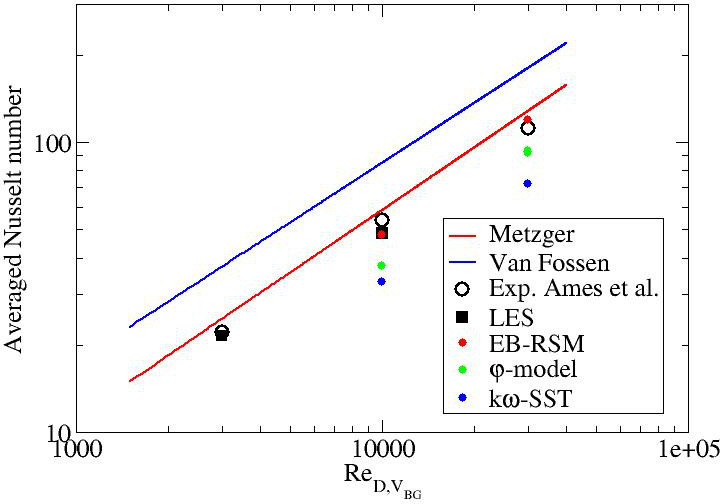Figure 26: Average Nusselt number on the bottom wall

### Local comparisons

#### Mean pressure coefficient

Figures 27 to 29 give the profiles of the pressure coefficient along the midline of pins 1, 2, 3 and 5 for the three Reynolds numbers.

Note that the experimental results have an uncertainty of +/-0.075 at $Re_{D}=3000$and of +/-0.025 at $Re_{D}=10000$and $Re_{D}=30000$.

LES results are in relatively good agreement with the experimental data. EB-RSM exhibits decent results as well. The two eddy viscosity models show poor results in particular the φ-model. The prediction of the $C_{p}$coefficient may be related to the wake prediction downstream of the pins.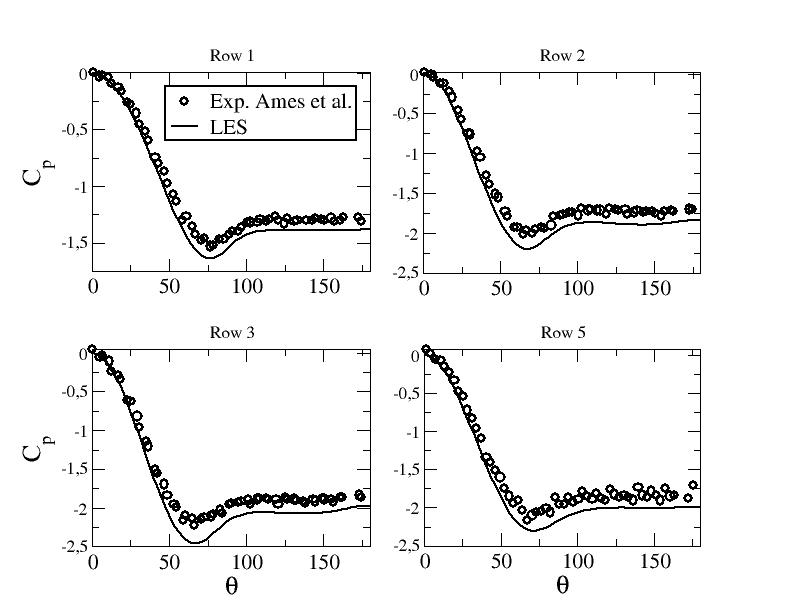Figure 27: Pressure coefficient along the midline of the pins - LES at $Re_{D}=3000$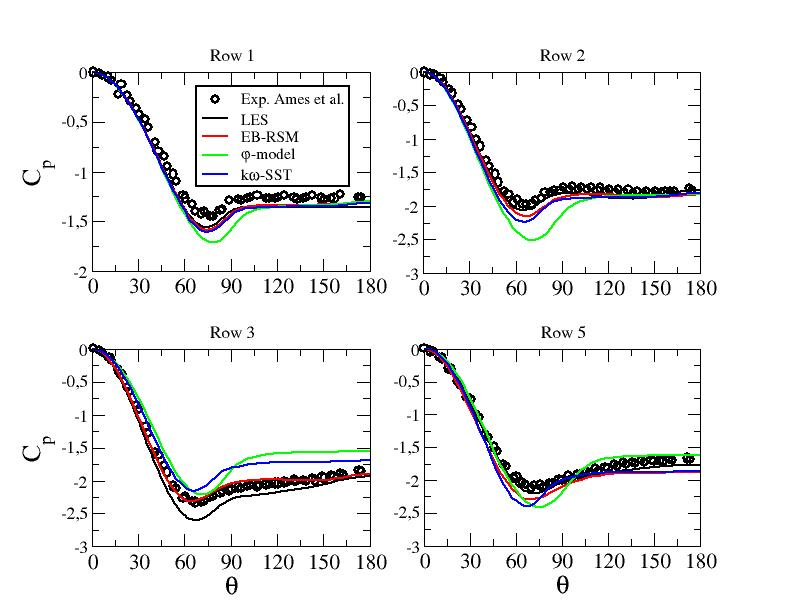Figure 28: Pressure coefficient along the midline of the pins - LES and URANS computations at $Re_{D}=10000$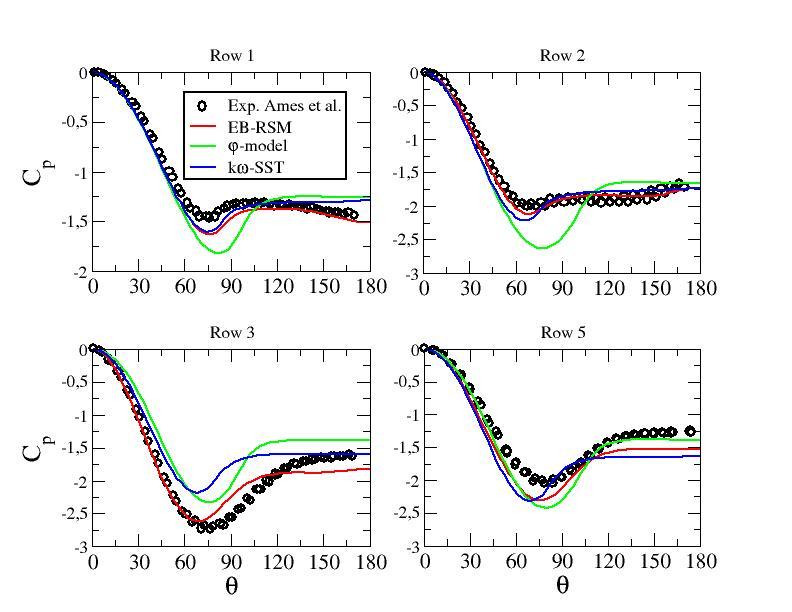Figure 29: Pressure coefficient along the midline of the pins - URANS computations at $Re_{D}=30000$#### Mean velocity profiles and contours

Figures 30 to 32 show the mean axial velocity component along line B for the different computations. The two eddy viscosity models exhibit a dramatic deficit of the mean axial velocity. On the other hand, LES and EB-RSM perform well compared to the experimental data with a slight overestimation of the mean stream-wise velocity peaks close to the walls in several locations, in particular departing from row 3. Note that the convergence study shown in the "Test Case Studies" section proved that the needed refinement to obtain a convergent solution around pin 2 is very important (with the first two levels of refinement, a deficit in the central velocity was observed).

Figure 33 shows the means axial velocity component along line A1 for the highest Reynolds number. One can see again that the linear eddy viscosity models are unable to predict the present flow. The results obtained with the EB-RSM model are much closer to the experimental data. However, the flow redistribution doesn't seem to be similar to the one obtained experimentally. This may come from the periodic boundary conditions used in the present work v.s. confined conditions in the experiment.

Figures 34 and 35 display the dimensional mean stream-wise velocity field in the midplane (Z=H). The wakes downstream of the cylinders are similar in LES and EB-RSM. The wakes are shorter downstream of the cylinders from row 4. Longer wakes are obtained for rows 1 and 2, then the lengths decrease. This is not observed at all for the φ-model and the kω-SST model. One can state at this stage that eddy viscosity models are not able to predict the present flow. The values obtained with these two models are still plotted, but without analyzing deeply the results.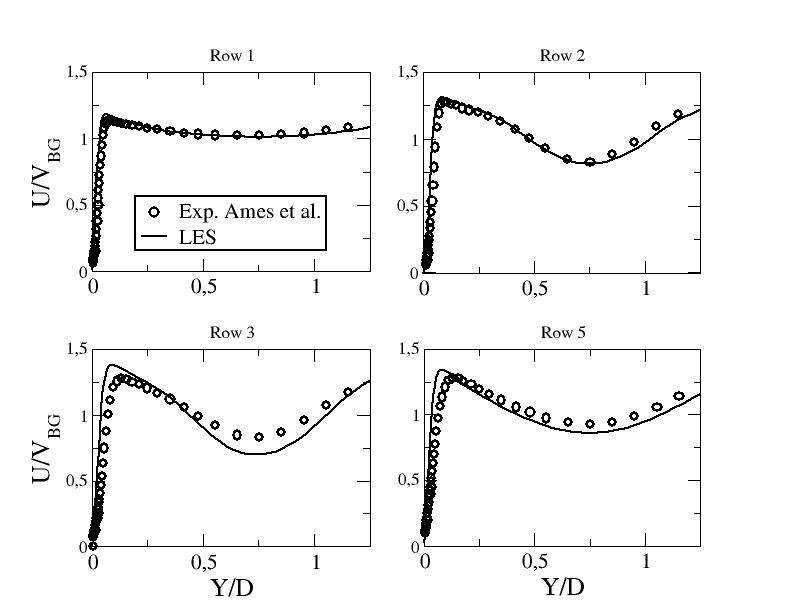Figure 30: Mean stream-wise velocity component along line B - LES at $Re_{D}=3000$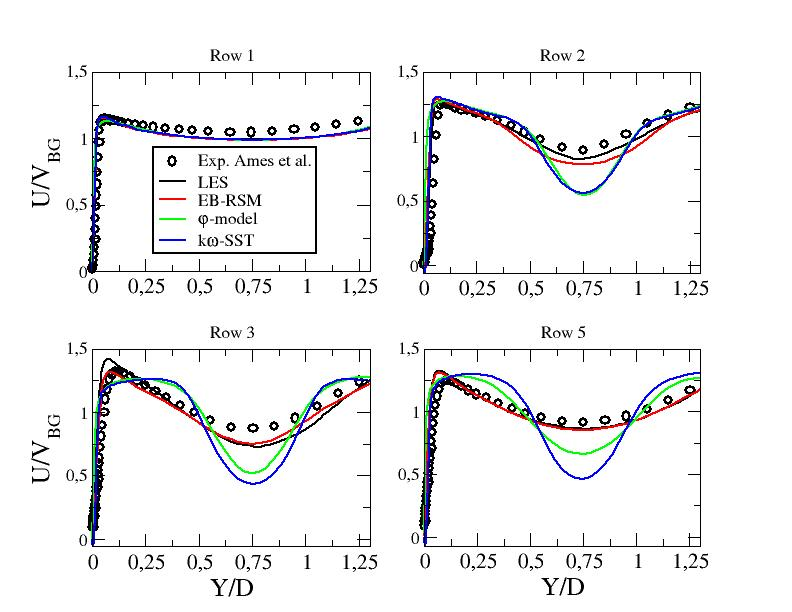Figure 31: Mean stream-wise velocity component along line B - LES and URANS computations at $Re_{D}=10000$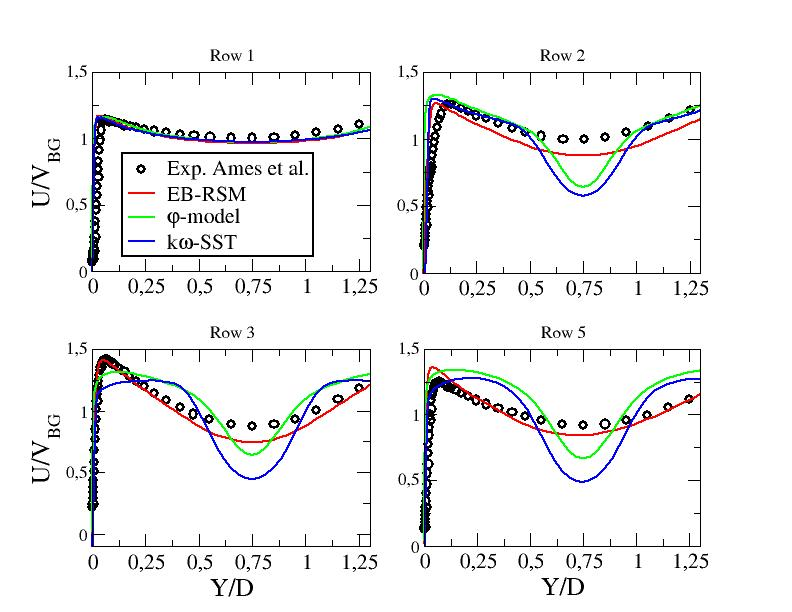Figure 32: Mean stream-wise velocity component along line B - URANS computations at $Re_{D}=30000$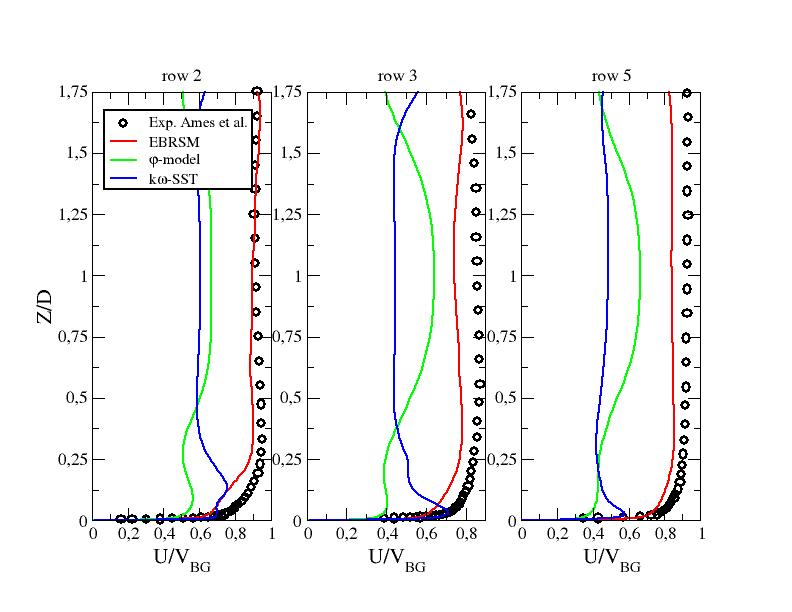Figure 33: Mean stream-wise velocity component along line A1 - URANS computations at $Re_{D}=30000$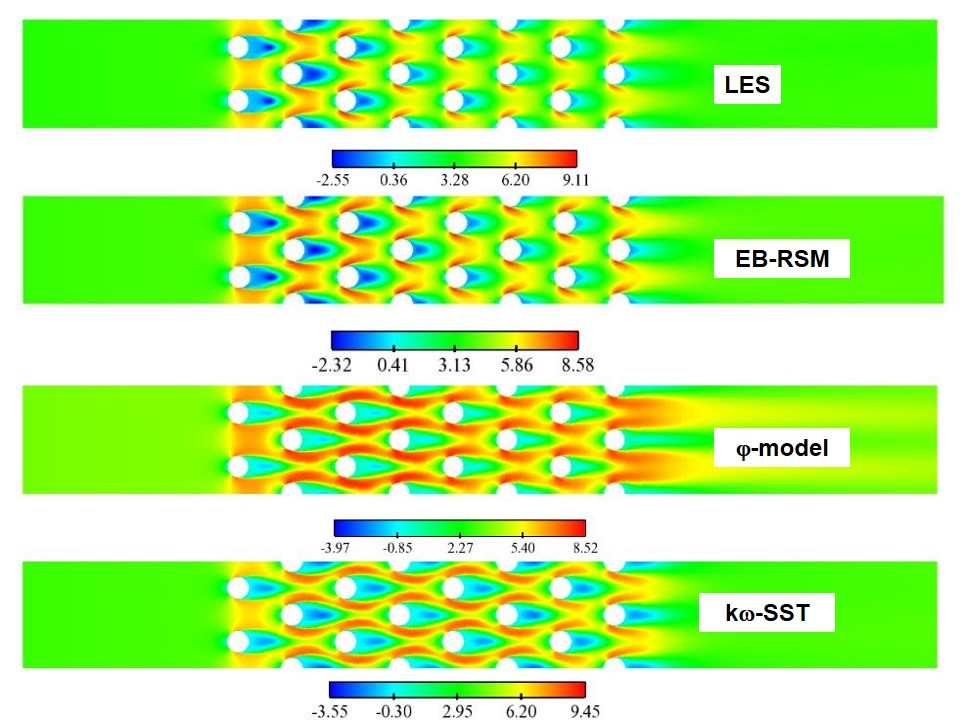Figure 34: Dimensional mean stream-wise velocity (m/s) in the midplane z=H at $Re_{D}=10000$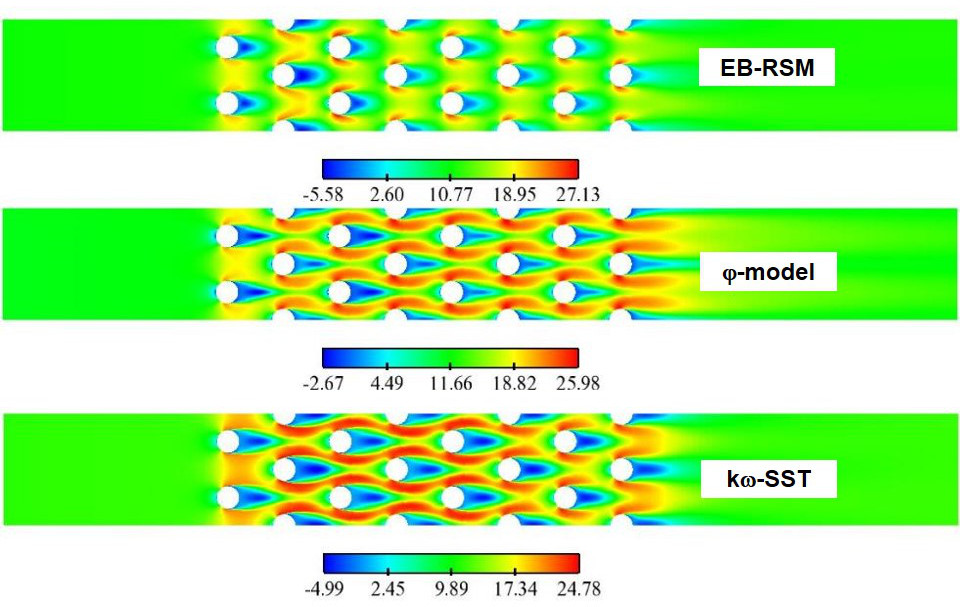Figure 35: Dimensional mean stream-wise velocity (m/s)in the midplane z=H at $Re_{D}=30000$#### R.m.s. velocity profiles

Figures 36 to 38 and figure 39 show the r.m.s. value of the stream-wise velocity along line B and line A1, respectively. The eddy viscosity models are very far from the experimental measurements (of course one cannot expect linear eddy viscosity models to yield realistic normal stresses, i.e. u', but only the sum k). On the other hand, LES and EB-RSM perform well compared to the experimental data with a certain superiority of LES. One can notice an overestimation of the r.m.s. value of the stream-wise velocity component with the EB-RSM model in the central part of row 5 (along both lines B and A1).

Figures 40 to 42 give the detailed profiles of the resolved and modelled stresses for the two Reynolds numbers at which the test case is performed with URANS approaches along lines B and A1, respectively. It is assumed here that the total Reynolds stress is given by the sum of the modelled part and the resolved one: $R_{ij}^{tot}={\overline {u'_{i}u'_{j}}}+{\overline {R}}_{ij}$, where $u'_{i}$is the velocity fluctuation (resolved part) and ${\overline {R}}_{ij}$the time averaged value of the modelled Reynolds stress ${R}_{ij}$. First, the EB-RSM model doesn't degenrate to a Large Eddy Simulation. The modelled part does't vanish and enhances the total level of turbulence. The linear eddy viscosity models fail naturally to predict the normal stresses. Figures 43 and 44 give the modelled and resolved stresses in the mid-plane in order to have a better overview of the behavior of this quantity (the whole computational domain is not represented to enhance clarity). The kω-SST model is clearly steady upstream of row 5 for both Reynolds numbers. The φ-model shows some unsteadiness starting from row 3 at Re=10000 but is totally steady at Re=30000. Note that at this Reynolds number, the φ-model exhibited unsteadiness everywhere with a coarser mesh. This is an exemple of a computation which might exhibit unsteadiness at relatively coarse meshes and gives a steady solution after refinement (see Fadai-Ghotbi et al. (2008)).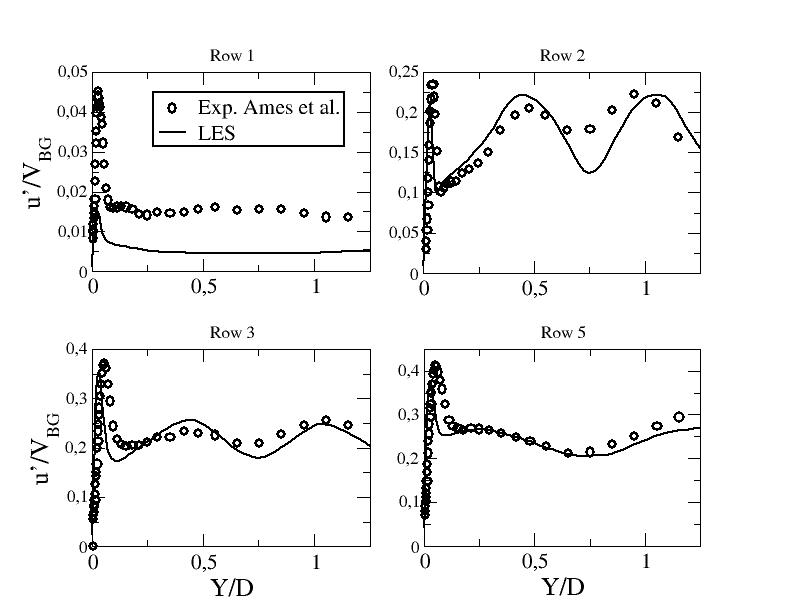Figure 36: R.m.s. of the stream-wise velocity component along line B - LES at $Re_{D}=3000$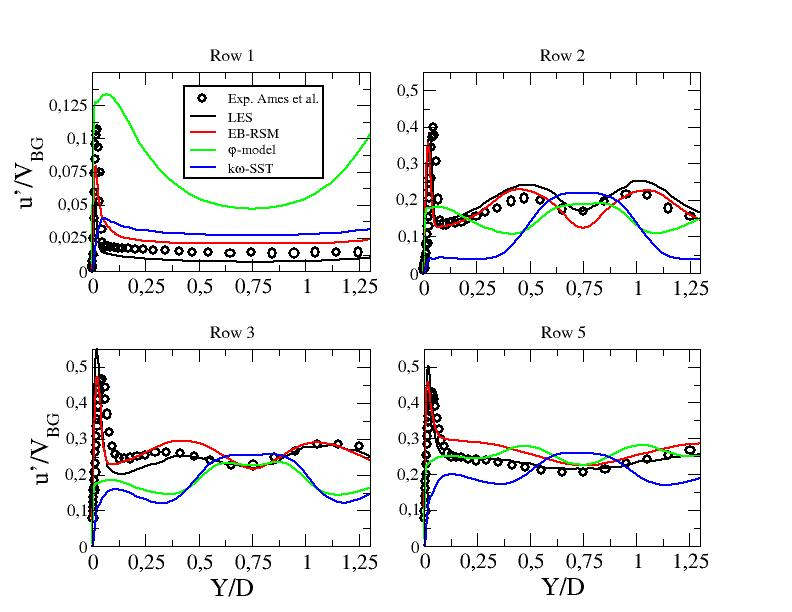Figure 37: R.m.s. of the stream-wise velocity component along line B - LES and URANS computations at $Re_{D}=10000$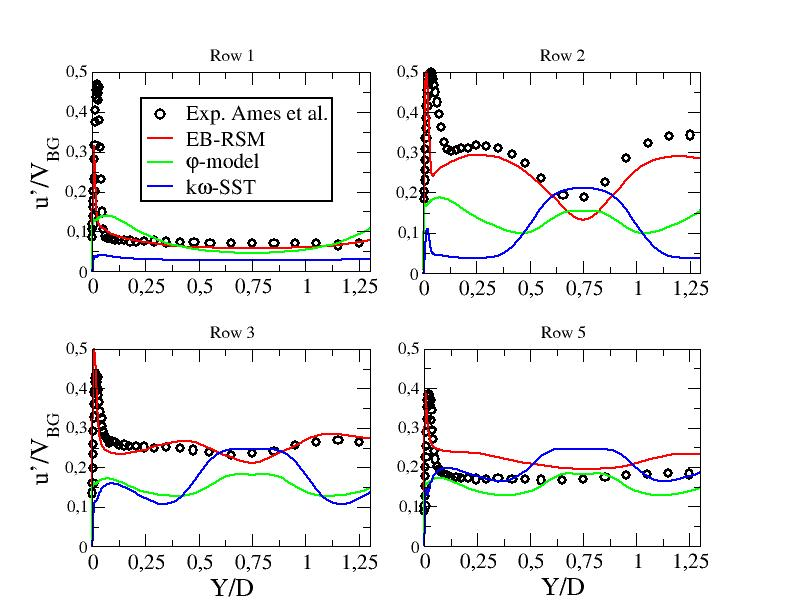Figure 38: R.m.s. of the stream-wise velocity component along line B - URANS computations at $Re_{D}=30000$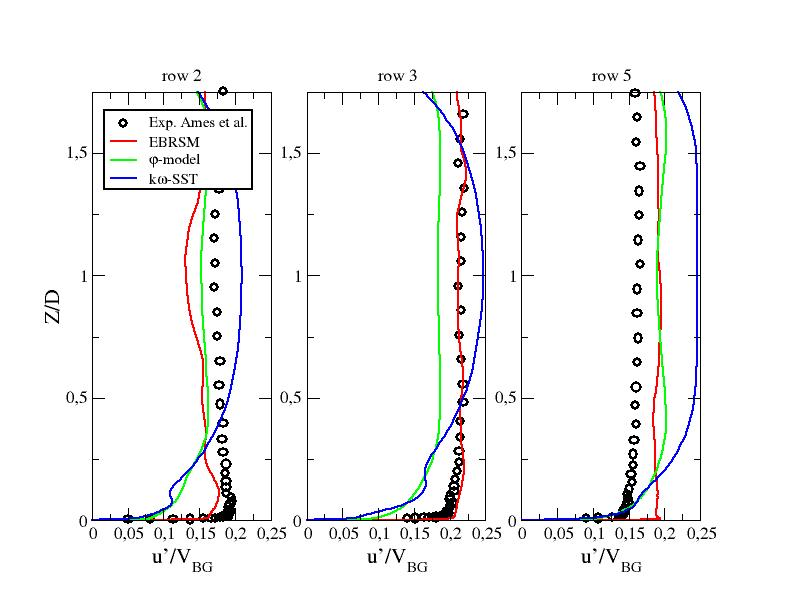Figure 39: R.m.s. of the stream-wise velocity component along line A1 - URANS computations at $Re_{D}=30000$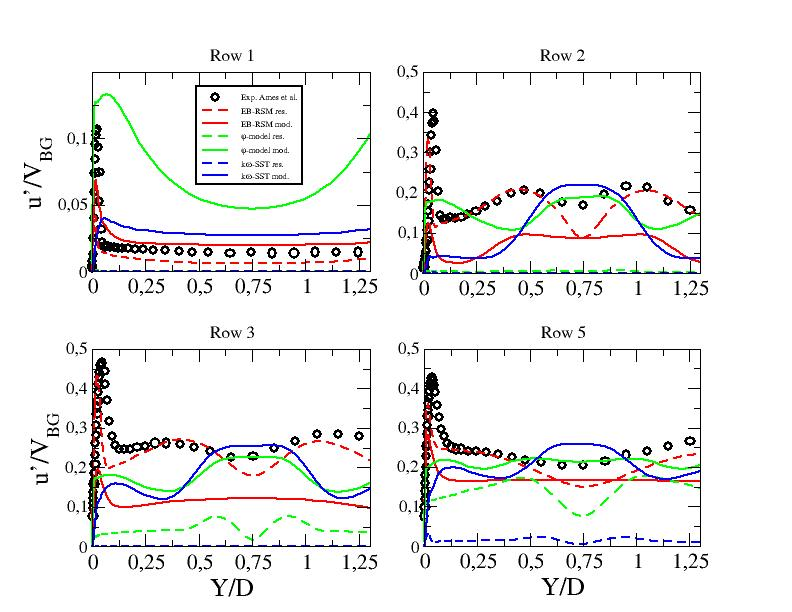Figure 40: Resolved and modelled contributions to the r.m.s. of the stream-wise velocity component along line B - URANS computations at $Re_{D}=10000$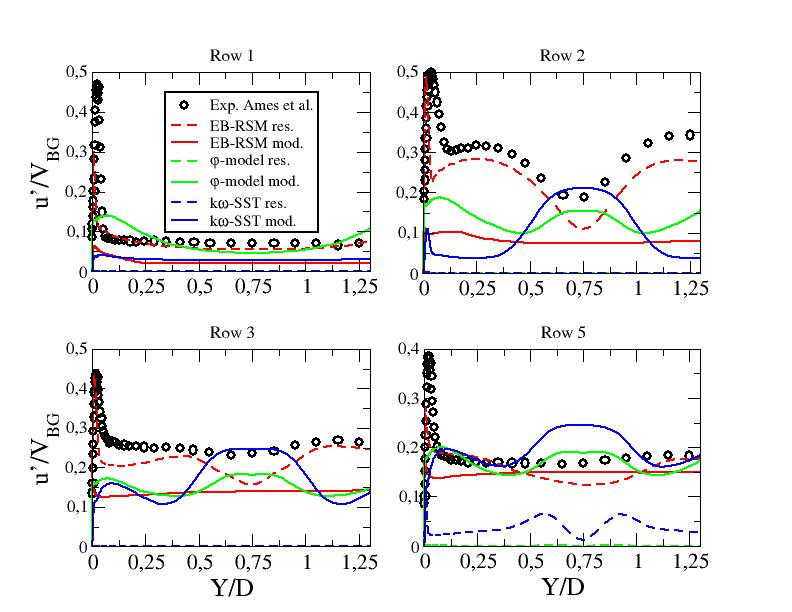Figure 41: Resolved and modelled contributions to the r.m.s. of the stream-wise velocity component along line B - URANS computations at $Re_{D}=30000$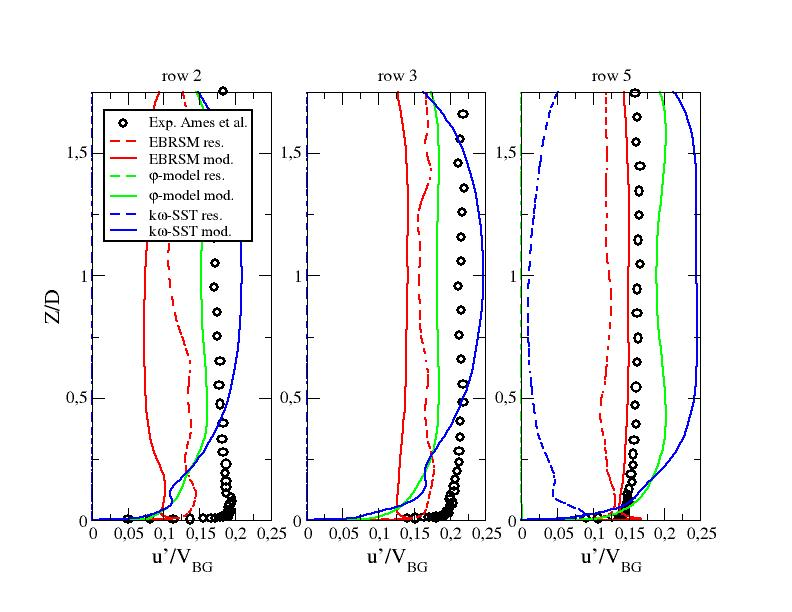Figure 42: Resolved and modelled contributions to the r.m.s. of the stream-wise velocity component along line A1 - URANS computations at $Re_{D}=30000$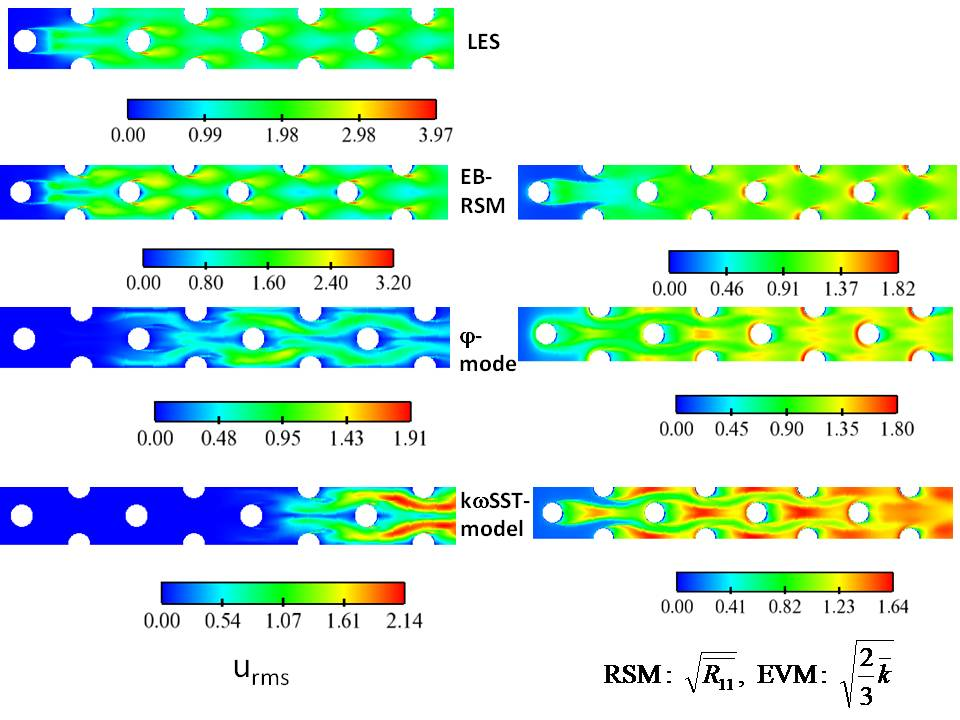Figure 43: Resolved (left) and modelled (right) contributions to the dimensional r.m.s. of the stream-wise velocity component (m/s) in the midplane - LES and URANS computations at $Re_{D}=10000$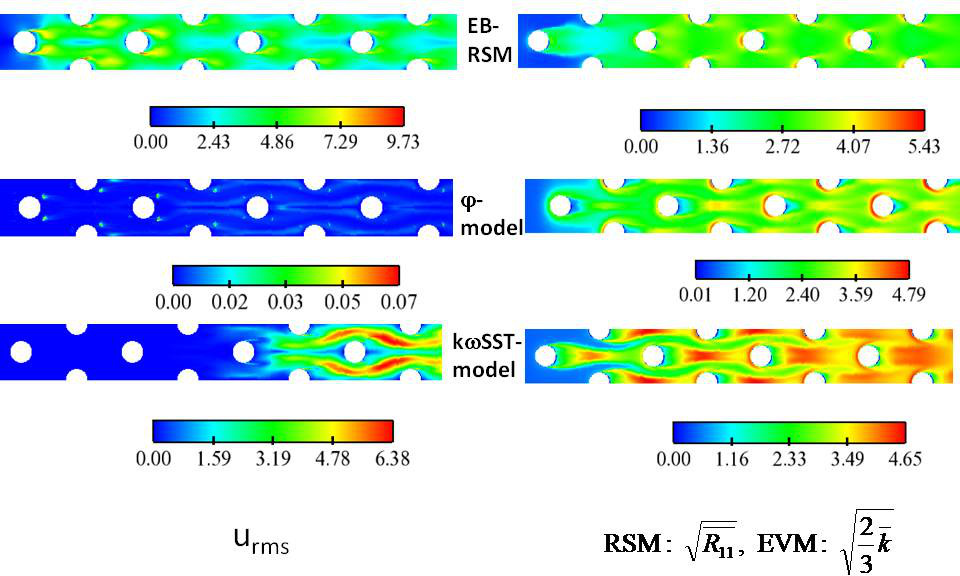Figure 44: Resolved (left) and modelled (right) contributions to the dimensional r.m.s. of the stream-wise velocity component (m/s) in the midplane - URANS computations at $Re_{D}=30000$#### Nusselt number on the bottom wall

Figures 45 to 47 show contours of the Nusselt number normalized by its averaged value over the bottom wall for the 8 rows.

LES computations exhibit an excellent behaviour compared to experimental values. The Nusselt number seems however overestimated at the locations of the horseshoe vortices and underestimated in the wake of the third row, in particular at the highest Reynolds number. Recall here that the error made on the average of the normalized Nusselt number is equal to 3% and 1% for $Re=3000$and $Re=10000$, respectively.

The φ-model and the kω-SST model are inapt for predicting the physics and thus local quantities such as the Nusselt number.

The EB-RSM model exhibits a very satisfactory global behaviour in particular at the highest Reynolds number. The Nusselt number is however underestimated in the wake of the first cylinder as the unsteadiness is lower than predicted by LES. The prediction of the next rows is satisfactory although an overestimation of heat transfer in the wake of the cylinders for the last 4 rows is observed.

Figures 48 to 50 show detailed profiles of the normalized Nusselt number along particular lines (see "Test Case Studies" section). LES exhibits excellent results in particular after the second pin. The EB-RSM model shows some discrepencies for $Re=10000$and very good results at $Re=30000$in particular starting from row 5 (where the statistically periodic behavior is supposed to be reached).Figure 45: Local Nusselt number normalized by its average ($Nus/Nus_{av}$) on the bottom wall - LES computation at $Re_{D}=3000$vs. experimental results from Ames et al.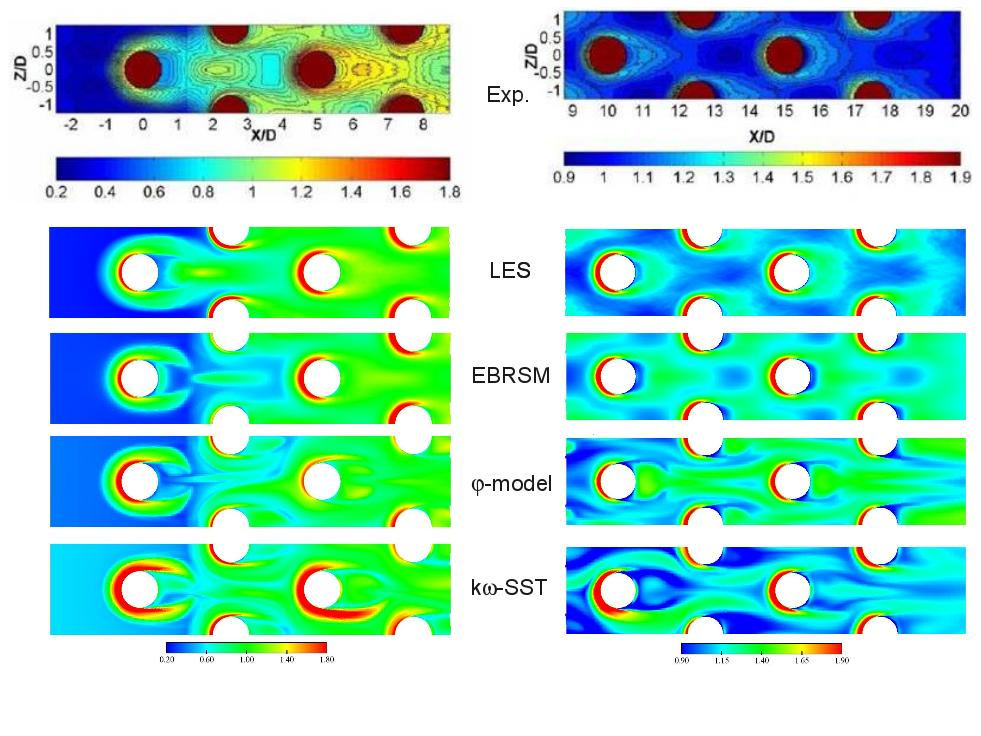Figure 46: Local Nusselt number normalized by its average ($Nus/Nus_{av}$) on the bottom wall - LES and URANS computations at $Re_{D}=10000$vs. experimental results from Ames et al.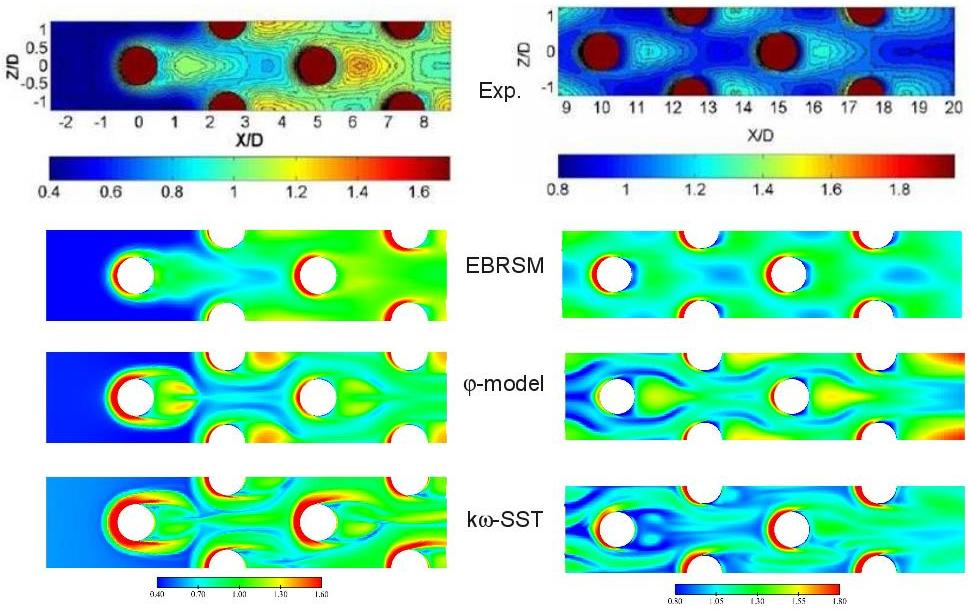Figure 47: Local Nusselt number normalized by its average ($Nus/Nus_{av}$) on the bottom wall - URANS computations at $Re_{D}=30000$vs. experimental results from Ames et al.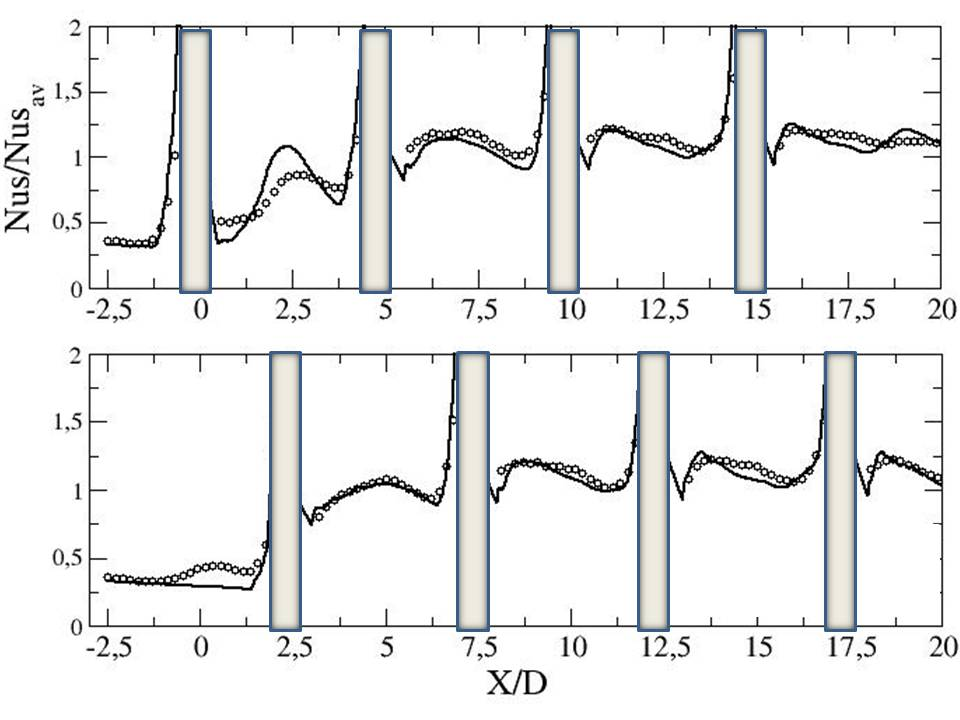Figure 48: Local Nusselt number normalized by its average ($Nus/Nus_{av}$) - $Re_{D}=3000$- top: Y=0, bottom: Y=1.25 - symb.: Exp. - black: LESFigure 49: Local Nusselt number normalized by its average ($Nus/Nus_{av}$) on the bottom wall - $Re_{D}=10000$- top: Y=0, bottom: Y=1.25 - symb.: Exp. - black: LES - red: EBRSM - blue: Phi-model - green: kw-SST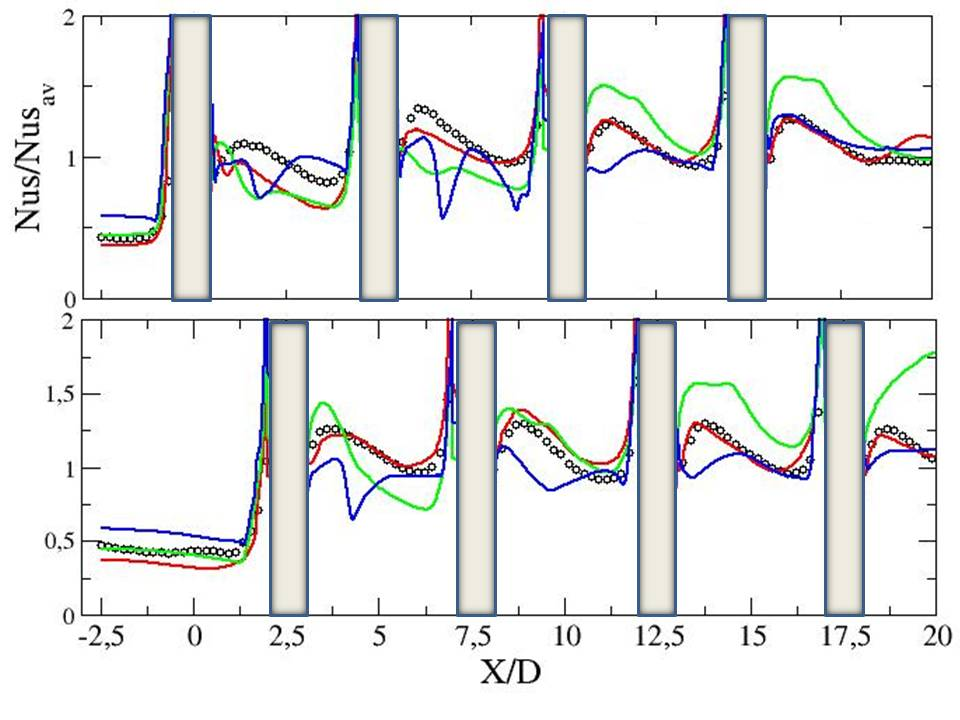Figure 50: Local Nusselt number normalized by its average ($Nus/Nus_{av}$) on the bottom wall - $Re_{D}=30000$- top: Y=0, bottom: Y=1.25 - symb.: Exp. - red: EBRSM - blue: Phi-model - green: kw-SST

Contributed by: Sofiane Benhamadouche — EDF